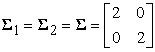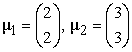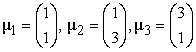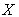﻿ Comparison of Single and Ensemble Classifiers of Support Vector Machine and Classification Tree

### Comparison of Single and Ensemble Classifiers of Support Vector Machine and Classification Tree

Iut Tri Utami, Bagus Sartono, Kusman SadikOPEN ACCESSPEER-REVIEWED

## Comparison of Single and Ensemble Classifiers of Support Vector Machine and Classification Tree

Iut Tri Utami1,, Bagus Sartono2, Kusman Sadik2

1Department of Mathematics, Tadulako University, Palu, Indonesia

2Department of Statistics, Bogor Agricultural University, Bogor, Indonesia

### Abstract

An ensemble consists of a set of individually trained classifiers (such as Support Vector Machine and Classification Tree) whose predictions are combined by an algorithm. Ensemble methods is expected to improve the predictive performance of classifier. This research aims to assess and compare performance of single and ensemble classifiers of Support Vector Machine (SVM) and Classification Tree (CT) by using simulation data. The simulation data is based on three data structures which are linearly separable, linearly nonseparable and nonlinearly separable data. The simulation data results show that SVM has the ability to classify the data better than CT. Ensemble method improve classification performance and more stable than single classifier. This was due to ensemble SVM has the smallest percentage of the average misclassification rate and standard deviation.

### At a glance: Figures

1
Prev Next

• Utami, Iut Tri, Bagus Sartono, and Kusman Sadik. "Comparison of Single and Ensemble Classifiers of Support Vector Machine and Classification Tree." Journal of Mathematical Sciences and Applications 2.2 (2014): 17-20.
• Utami, I. T. , Sartono, B. , & Sadik, K. (2014). Comparison of Single and Ensemble Classifiers of Support Vector Machine and Classification Tree. Journal of Mathematical Sciences and Applications, 2(2), 17-20.
• Utami, Iut Tri, Bagus Sartono, and Kusman Sadik. "Comparison of Single and Ensemble Classifiers of Support Vector Machine and Classification Tree." Journal of Mathematical Sciences and Applications 2, no. 2 (2014): 17-20.

 Import into BibTeX Import into EndNote Import into RefMan Import into RefWorks

### 1. Introduction

A classifier is such a rule that can be used to group an object into predetermined group or classs based on its attributes. There are two types of approach to develop a classifier rules: a parametric approach; and a nonparametric approach. Linear Discriminant Analysis (LDA) and Logistic Regression (LR) are two parametric classifiers which have been extensively used in classification problems. Implementation of those methods requires some restrictive assumptions such as the linearity, normality and independence among predictor variables. The violation of the assumptions might lead to the lack of the effectiveness and the validity results. Recently, people pay more attention to non parametric classifiers such as Support Vector Machine (SVM) and Classification Tree (CT) since its flexibility on the data requirement and its excelent empirical performance.

Moreover, some resent research figured out that an ensemble of classifiers could be an effective way to improve the classification accuracy and reduce the prediction variation of a single classifier . The basic idea of the ensemble method is to combine the class predictions resulted by a set of single classifiers into a single prediction by applying a majority vote rule. Among some popular techniques a method of bagging (bootstrap agregating) is the simplest but powerful technique .

Researches on ensemble classification with various base classifiers has been carried out by several authors [1, 5, 6, 7, 9]. While many papers studied based on real-life data, we did simulation research to assess the performance of bagging ensembles of CT and SVM. We worked with three different data structure to examine the impact of doing ensemble compared to the result of a single classifier. By this study we compare also the performance of ensemble-tree and ensemble-SVM, in term of the ability to provide low missclassification rate and the stability of that rate.

The structure of the paper can be mentioned as follow. At Section 2 we explained the simulation procedure we performed, including the parameters of the generated data. Next at Section 3 we presented the general results and provided discussion on those. We enclose the paper by small conclusion.

### 2. Simulation Setting

The performance comparison of the prediction quality of a single classifier and its ensembles was done by involving three different scenarios of data structure. We decided to examine the performance for the following structures : (1) a situation where the members of different classes are perfectly linear separable, (2) a situation where the members of different classes are liner-separable but not perfect, and (3) a situation where the members of different classes could not be separated by a linear function. We limit ourselves to work with data having two classes and containing two explanatory variables.

In the simulation data we have a data set with size 1200 2, containing 2-dimensional feature vectors describing 1200 objects. We would like to use data to build the classifier (training data), and also to build test its performance (testing data). The training data was done by randomly generating a sets offrom the original data set, with replacement. The data set was randomly split into 70% was used for training a linear classifier, and the other was used for testing. Then we assess and average the misclassification rate and standard deviation value of the classifiers built on these sets.

The simulation data for a two class consists of objects labeled with one of two labels corresponding to the two classes; we assume the labels are +1 (positive examples) or -1 (negative examples). To illustrate the first scenario, we use simulated data from a normal mixture distribution consisting of two components as a positive and negative examples with mean vector of each isand a common covariance matrixThe second scenario also arises from assuming the observations in group positive examples and those in group negative examples have a multivariate distribution with mean vector of each isand common covariance matrixSuppose we have a random sample of 1200 observations from these scenario with 600 observations in each group. The third scenario was generated by a mixture of three different distribution with mean vector of each isand common covariance matrixwith 40 observations as a positive examples and 80 observations as a negative samples.

A brief description of our simulation could be represented by Fig. 1. First we generated a data set with one of aforementioned three pattern. Then, the data set was then used as an input of two different approach, single and ensemble classifier. We implemented a bagging classifier throughout the simulation. For every data set we recorded the missclassification rate of the resulted classifier rules. Those steps were then repeated 5000 times so that we were able to calculate two statistics of the misclassification rate values which are the average and the standard deviation.

Within this study we applied CT and SVM as the classifier method so that in total we have four approach to be compared: single tree, ensemble-tree, single-SVM, ensemble-SVM. The bootstrap part of the ensemble approach were perform by doing resampling step as many as 50, 100, and 500 times. We used those resamplig procedure to recognize the effect of resampling frequency to the classification performance. Simulations was carried out with two conditions of bootstrap sample size and training data: (1) the conditions are the same bootstrap sample size to training data and (2) the bootstrap sample size is smaller than the training data.

In the implementation of SVM, it is worthy to implement a kernel trick to handle non-linear separation. The kernel trick in principle is a kind of transformation from low-dimensional space into high-dimensional space so that the existing classes can be linearly separated easier. There are three different kernel transformation that we included: linear, polynomial and radial .

Tree structured classifiers or binary tree structured classifiers are constructed by repeated splits of subsets ofinto two descendant subsets, beginning withitself . CT is built in accordance with splitting rule that performs the splitting of learning sample into smaller parts. The basic idea is to select each split of a subset so that the data in each of the descentdant subsets are purer than the data in the parent subset. The node impurity is largest when all classes are equally mixed together in it, and smallest when it contains only one class.

#### Table 1. Confussion Matrix

Performance of single and ensemble classifiers can be calculated by confussion matrix by using the testing data set. It contains the number of elements that have been correctly or incorrectly classified for each class. For every instance in the test set will be compared the actual class to the class that was assigned by the trained classifier. These numbers can be organized in a confusion matrix as shown in Table 1.

Based on the values in Table 1, one can calculate all the measures defined above:

Accuracy is: (a+d)/n

Misclassification rate is: (b+c)/n

Precision is: d/ n0

True positive rate (Recall) is: d/n0

True negative rate (Specificity) is: a/ n1

Illustration of simulation data from three data structures are shown in Figure 2.

### 3. Results

Simulation data results obtained in both conditions bootstrap and training data sample size are very similar so that the simulation results will be shown with bootstrap sample size is smaller than the size of the training data samples. The results of simulation data of single and ensemble classifiers in the three data structures are shown in Table 2. Table 2 gives the percentage of average and standard deviation misclassification rate of the single and ensemble classifier applied on three data structures with repeated 5000 times.

The results in Table 2 show that for three data structures, single SVM achieved better prediction accuracy comparing to CT. This can be seen in the single classifiers, the percentage of average misclassification rate of SVM was smaller than CT. Standard deviation value of SVM also performed smaller than CT so that SVM is more stable classifier than CT.

The percentage of average missclassification rates achieved by both ensemble classifiers are very similar. Both ensemble classifiers more decline as more bagging and iterations performed. However, the ensemble-SVM achieved slightly lower missclassification rates for all data structures. In overall ensemble classifiers able to improve performance of classification both SVM and CT. Standard deviation values on the ensemble classifiers is smaller than the single classifiers so that the ensemble classifiers provide more stable performance than a single classifier.

### 4. Conclusions

In this paper, assessment and comparison about single and ensemble classifiers using simulation data are presented. The results can be summarized as follows. Simulations were performed on three data structures with two conditions bootstrap sample size and training data obtained similar results. First, single SVM can classify the data better than CT. This is evident from the percentage of average misclassification rate of SVM is smaller than CT. Second, the performance of ensemble classifiers is improved by learning an ensemble (using CT and SVM). Third, ensemble classifiers provide a more stable performance and reduce standard deviation of a single classifier.

### References

  Breiman, L., “Bagging Predictors,” Machine Learning, 24. 123-140. 1996.In article CrossRef  Breiman, L., Friedman, J.H., Olshen, R.A. and Stone, C.J, Classification and Regression Trees, Chapman and Hall, New York, 1993.In article  Cortes, C. and Vapnik, V., “Support-Vector Networks,” Machine Learning 20(3). 273-297. 1995.In article CrossRef  Cristianini, N. and Shawe-Taylor, J., An introduction to Support Vector Machines and other kernel-based learning methods, Cambridge University Press, 2000. [E-book] Available: libgen.org.In article  Dietterich, T.G., “An experimental comparison of three methods for constucting ensembles of decision trees: Bagging, boosting and randomization,” Machine Learning, 40 (2). 139-158. 2000.In article CrossRef  Hansen, L.K. & Salamon, P., “Neural Network Ensembles,” IEEE Trans. Pattern Analysis and Machine Intelligence, 12(10): 993-1001. 1990.In article CrossRef  Opitz, D. and Maclin, R., “Popular Ensemble Methods: An Empirical Study,” Journal Of Articial Intelligence Research, 11. 169-198. 1999.In article  Valentini, G. and Dietterich, T.G., “Bias–variance analysis of Support Vector Machines for the development of SVM-based ensemble methods,” Journal of Machine Learning Research, 1. 1-48. 2000.In article  Wang, S.J., Mathew, A., Chen, Y., Xi, L.F., Ma, L. And Lee, J., “Empirical analysis of support vector machine ensemble classifiers,” Expert Systems with Applications, 36. 6466–6476. 2009.In article CrossRef